### Lagrangian problems, constrained point masses

#### Problem:

A circular hoop of radius r rotates with angular frequency ω about a vertical axis through the center of the hoop in the plane of the hoop.  A bead of mass m slides without friction around the hoop and is subject to gravity.
(a)  Give the Lagrangian in terms of the angle θ shown in the drawing.
(b)  Find the equation of motion in terms of this angle.
(c)  Find the values of θ for which the bead may be stationary with respect to the hoop and determine which of the stationary points are stable.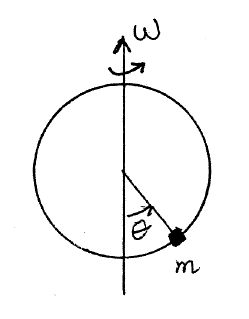Solution:

• Concepts:
Lagrange's equations
• Reasoning:
Lagrange's equations are the equations of motion.
• Details of the calculation:
(a)  L = T - U.
T = ½m(r2(dθ/dt)2 + r2sin2θ ω2),  U = -mgr cosθ.
L =  ½m(r2(dθ/dt)2 + r2sin2θ ω2) + mgr cosθ.
Here r and ω are constants.
(b)  d/dt(∂L/∂(dqi/dt)) -  ∂L/∂qi = 0.
∂L/∂(dθ/dt) = mr2(dθ/dt),  ∂L/∂θ = mr2sinθ cosθ ω2 - mgr sinθ.
mr2d2θ/dt2 - mr2sinθ cosθ ω2 + mgr sinθ = 0.
d2θ/dt2 = sinθ cosθ ω2 - (g/r) sinθ.
(c)  d2θ/dt2|θequ = 0 for a stationary point.
The angles θ = 0, θ = π, or cosθ  = g/(rω2) define stationary points.
We need g/(rω2) < 1 for the point θ = cos-1(g/(rω2) to exist.

For a stable point we need a restoring force.  Let θ = θequ + δ.
We need d2δ/dt2 = -cδ, with c a positive number.
d2δ/dt2  = sin(θequ + δ)cos(θequ + δ) ω2 - (g/r) sin(θequ + δ)
= d(sinθ cosθ ω2 - (g/r) sinθ)/dθ|θequ*δ  (Taylor series expansion)
= (cos2θqequ ω2 - sin2θθequ ω2 - (g/r) cosθθequ)δ.
For a stable point we need cos2θθequ ω2 - sin2θθequ ω2 - (g/r) cosθθequ to be negative.
Check:
For the stationary point θθequ = 0:
cos2θθequ ω2 - sin2θθequ ω2 - (g/r)cos θθequ = ω2 - (g/r).
If g/r > ω2  then this point is stable, otherwise it is unstable.
For the stationary point θθequ = π:
cos2θθequ ω2 - sin2θθequ ω2 - (g/r)cosθθequ = ω2 + (g/r).
This point is always unstable.
For the stationary point θθequ = cos-1(g/(rω2)):
cos2θθequ ω2 - sin2θθequ ω2 - (g/r)cosθθequ = g2/(r2ω2) - ω2 < 0 since g/(rω2) < 1 for the point to exist.
This point is always stable if it exists.

Similar problems

#### Problem: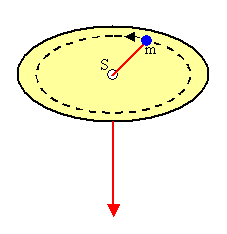In the figure above the mass point m rotates about the point s.  At the same time the thread holding the mass point is shortened continuously.  Find the constants of motion.

Solution:

• Concepts:
Lagrange's Equations
• Reasoning:
All forces except the forces of constraint are derivable from a potential.  To find the constants of motion we find the Lagrangian and look for cyclic coordinates.  If the generalized coordinates and momenta we choose do not depend on time and the Lagrangian depends explicitly on time, then the energy is not conserved.
• Details of the calculation:
Let x and y be the Cartesian coordinates in the plane of the circle and r and Ф be the polar coordinates.
r = R0 - ct is equation of constraint.
The constraint is holonomic and time dependent.
x  = (R0 - ct)cosФ, y = (R0 - ct)sinФ.
T = ½m(vx2 + vy2) = ½m((dФ/dt)2(R0 - ct)2 + c2).
L = T,  we have only one generalized coordinate, Ф, which does not explicitly depend on time.
∂L/∂Ф = 0,  Ф is cyclic.
∂L/∂(dФ/dt) = m(dФ/dt)(R0 - ct)2 = constant, since  (d/dt)(∂L/∂(dФ/dt)) = 0.
The angular momentum about the vertical axis is conserved.
H = H(t) = T(t).  H explicitly depends on time.  The energy is not a constant of motion.

#### Problem:

An object of mass m slides on a horizontal, friction-free horizontal table.  A light, inextensible string, which passes through a small hole in the table, attaches the mass to a second body of mass M.  The second body hangs below the table where g = -9.8 m/s2 (see the sketch).  At time t = 0, mass m is located at position (r, θ).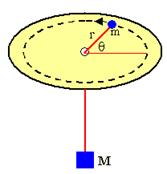(a)  Determine the differential equations governing the motion of the system.
(b)  For the special case that r = constant, solve the resulting equations and interpret your results.
(c)  What are the constants of motion for this system?

Solution:

• Concepts:
Lagrange's equations
• Reasoning:
All forces except the forces of constraint are derivable from a potential.  To find the constants of motion we find the Lagrangian and look for cyclic coordinates.  If the generalized coordinates and momenta we choose do not depend on time and the Lagrangian does not depend explicitly on time, then the energy is a constant of motion.
• Details of the calculation:
(a)  T = ½mr2(dθ/dt)2 + ½(M + m)(dr/dt)2,   U = Mgr + U0.  U0 is an arbitrary constant.
L = T - U,  ∂L/∂θ = 0, the coordinate θ cyclic, ∂L/∂(dθ/dt) = is a constant of motion.
∂L/∂(dθ/dt)  = mr2(dθ/dt) = C.
Energy conservation leads to ½(M + m)(dr/dt)2 + C2/(2mr2) + Mgr + U0 = E.
The first-order differential equations governing the motion of the system are
½(M + m)(dr/dt)2 + C2/(2mr2) - Mg(L - r) = E,   mr2dθ/dt = C.
The second-order differential equations governing the motion of the system are
∂(mr2(dθ/dt))/∂t = 0,   (M + m)d2r/dt2 - C2/(mr3) + Mg = 0.
(b)  r = constant = r0,  dr/dt = 0.
dθ/dt = ω = C/mr02, the angular velocity is constant.
E = ½m(r0ω)2 + Mgr0 + U0 = ½m(r0ω)2 = ½mv2, if we choose U0 = -Mgr0.
E is the kinetic energy for the circular motion.
Mg = C2/(mr03) = mv2/r0, Mg is the force responsible for the centripetal acceleration.
(c)  The angular momentum about the vertical axis is a constant of motion.
The energy E is a constant of motion.

#### Problem:

A rigid wire shaped like an upside-down L is spinning about its vertical segment as shown in the figure.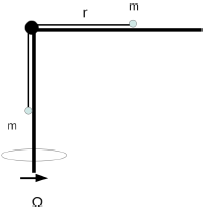The angular velocity of the motion is Ω.  A bead of mass m is constrained to slide without friction on the horizontal segment of the wire and is connected by a massless string to an identical bead on the vertical segment.  The string has constant length l and follows the shape of the wire without friction.  The bead on the vertical segment is subjected to gravity (g is downward).
Consider only situations when this geometry is valid.
(a)  In terms of the distance r of the revolving bead from the center of rotation, write down the Lagrangian for this system.
(b)  Find the equation of motion for the bead.
(c)  Solve the equation of motion for the trajectory of the bead.

Solution:

• Concepts:
Lagrangian Mechanics
• Reasoning:
We are asked to obtain Lagrange's equation of motion and solve them..
• Details of the calculation:
(a)  T = m(dr/dt)2 + ½mr2Ω2,  U = -mg(l - r).
L = T - U = m(dr/dt)2 + ½mr2Ω2 + mg(l - r).
(b)   We have only one generalized coordinate, r.
d/dt(∂L/∂(dr/dt)) - ∂L/∂r = 0.
∂L/∂(dr/dt) =  2m(dr/dt), d/dt(∂L/∂(dr/dt)) = 2m d2r/dt2.
∂L/∂r = mrΩ2 - mg.
d2r/dt2 = ½rΩ2 - ½g  is the equation of motion.
(c)  d2r/dt2 = ar - b.
Let r' = a(r - b/a), d2r'/dt2 = ar'.
r'(t) = C1exp((√a)t) +  C2exp(-(√a)t).
r(t) = C1exp((√a)t) +  C2exp(-(√a)t) + b/a.
The constants C1 and C2 are determined by the initial conditions.

#### Problem:

Consider the double pendulum consisting of two massless rods of length L = 1 m and two point particles of mass m = 1 kg in free space, with a fixed pivot point.
(a)  Write down the Lagrangian of the system shown in terms of the coordinates θ and α shown and the corresponding velocities.
(b)  Find two conserved quantities.
(c)  Write down the second-order differential equations of motion.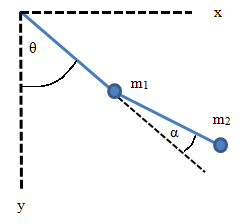Solution:

• Concepts:
Lagrangian mechanics
• Reasoning:
If one of the coordinates is cyclic, the conjugate momentum is conserved.
• Details of the calculation:
(a)  L = T = ½ v12 + ½ v22
x1 = sin(θ),   y1 = cos(θ),   x2 = sin(θ) + sin(θ + α),   y2 = cos(θ) + cos(θ + α)
v12 = (dθ/dt)2,
v22 = (dθ/dt)2 + (dθ/dt + dα/dt)2 + 2(dθ/dt)(dθ/dt + dα/dt)cos(α)
L = (dθ/dt)2 + ½(dθ/dt + dα/dt)2 + (dθ/dt)(dθ/dt + dα/dt)cos(α)
(b)  θ is cyclic, pθ = constant.
pθ = ∂L/∂((dθ /dt)) = 2(dθ/dt) + (dθ/dt + dα/dt) + (dθ/dt + dα/dt)cos(α) + (dθ/dt)cos(α)
= 3(dθ/dt) + (dα/dt) + 2(dθ/dt)cos(α) + (dα/dt)cos(α) = constant.
L = T = E does not explicitly depend on time.
E = (dθ/dt)2 + ½(dθ/dt + dα/dt)2 + (dθ/dt)(dθ/dt + dα/dt)cos(α) = constant.
(c) dpθ/dt = 0,  ∂L/∂((dα/dt)) -  ∂L/∂α = 0.
This yield the two second order equations of motion below.
(d2θ/dt2)(3 + 2 cos(α))  + (d2α/dt2)(1 + cos(α)) - 2(dθ/dt) (dα/dt)sin(α) - (dα/dt)2sin(α) = 0.
(d2θ/dt2) + (d2α/dt2)cos(α) + (dθ/dt)2 sin(α) = 0.

#### Problem:

A particle of mass equal to 3 kg moves in the xy plane.  The potential energy of the particle as a function of position is given by  U = (36 J/m2)xy - (48 J/m2)x2.  The particle starts at time t = 0 from rest at the point with position vector (x, y) = (10 m, 10 m).
(a)  Set up the differential equations describing the motion and solve them to determine the position of the particle as a function of time.
(b)  Find the velocity as a function of time.

Solution:

• Concepts:
Lagrange's Equations:  d/dt(∂L/∂(dq/dt)) -  ∂L/∂q = 0.
• Reasoning:
The potential energy is given.  All forces except the forces of constraint are derivable from a potential.  The Lagrangian formalism is well suited for such a system.
• Details of the calculation:
L = T - U = ½m((dx/dt)2 + (dy/dt)2) - 36xy + 48x2  (SI units).
∂L/∂(dx/dt) = m(dx/dt),  ∂L/∂(dy/dt) = m(dy/dt),  ∂L/∂x = -36y + 96x,  ∂L/∂y = -36x.
md2x/dt2 + 36y - 96x = 0,  md2y/dt2 + 36x = 0,  m = 3.
d2x/dt2 + 12y - 32x = 0,  d2y/dt2 + 12x = 0.
(a)  Let x = Aexp(at),  y = A'exp(at).
Then a2A +12A' - 32A = 0,  a2A' + 12A = 0, a2A - 144A/a2 -32A = 0.
a4 - 32a2 - 144 = 0,  a2 = 16 ± 20.
a2 = 36, a = ±6 yields exponential solutions.
a2 = -4, a = ±2i yields sinusoidal solutions.
If a2 = 36, then A' = -(1/3)A.
If a2 = -4, then A' = 3A.
The most general solution is a linear superposition of all possible solutions.
x = a1exp(6t) + a2exp(-6t) + a3cos(2t) + a4sin(2t),
y = -(a1/3)exp(6t) - (a2/3)exp(-6t) + 3a3cos(2t) + 3a4sin(2t).
dx/dt = 6a1exp(6t) - 6a2exp(-6t) - 2a3sin(2t) + 2a4cos(2t),
dy/dt = -2a1exp(6t) + 2a2exp(-6t) - 6a3sin(2t) + 6a4cos(2t).

At t = 0 we have (dx/dt) = 0, (dy/dt) = 0, 3a1 - 3a2 + a4 = 0, -a1 + a2 + 3a4 = 0.
At t = 0 we have x = 10, y = 10, a1 + a2 + a3 = 10, -a1 - a2 + 9a3 = 30.
Four equations for four unknowns yield a1 = 3, a2 = 3, a3 = 4, a4 = 0.
x(t) = 3exp(6t) + 3exp(-6t) + 4cos(2t) = 6cosh(6t) + 4cos(2t),
y(t) = -exp(6t) - exp(-6t) + 12cos(2t) = -2cosh(6t) + 12 cos(2t).
Below are contour and a surface plots of the potential for
-12.5 < x < 12.5,
-12.5 < y < 12.5.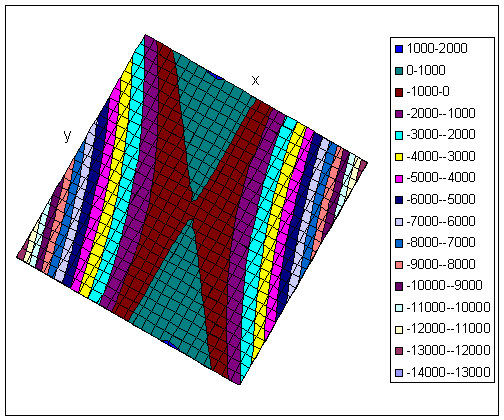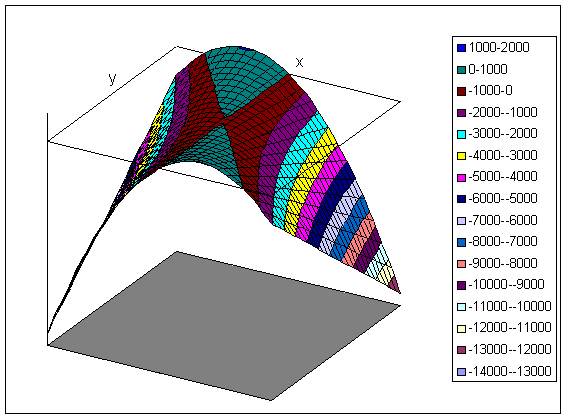(b)  dx/dt = 18exp(6t) - 18exp(-6t) - 8sin(2t) = 36 sinh(6t) - 8sin(2t).
dy/dt = -6exp(6t) + 6exp(-6t) - 24sin(2t) = -12sin

#### Problem:

Obtain Lagrange's equations of motion for a spherical pendulum (a mass point suspended by a rigid, weightless rod).

Solution:

• Concepts:
Lagrange's Equations: d/dt(∂L/∂(dqj/dt)) -  ∂L/∂qj = 0; generalized coordinates
• Reasoning:
We are asked to obtain Lagrange's equation of motion.  Let g point into the -z direction.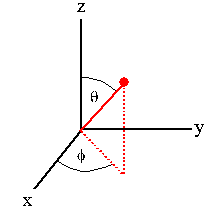The system has only two degrees of freedom.  Choose the generalized coordinates to be θ and Φ.  The constraints are holonomic, the applied forces are conservative.